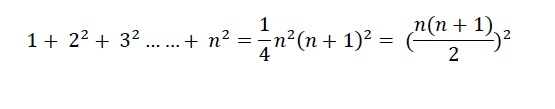# C Program for cube sum of first n natural numbers?

In this problem we will see how we can get the sum of cubes of first n natural numbers. Here we are using one for loop, that runs from 1 to n. In each step we are calculating cube of the term and then add it to the sum. This program takes O(n) time to complete. But if we want to solve this in O(1) or constant time, we can use this series formula −## Algorithm

### cubeNNatural(n)

begin
sum := 0
for i in range 1 to n, do
sum := sum + i^3
done
return sum
end

## Example

Live Demo

#include<stdio.h>
long cube_sum_n_natural(int n) {
long sum = 0;
int i;
for (i = 1; i <= n; i++) {
sum += i * i * i; //cube i and add it with sum
}
return sum;
}
main() {
int n;
printf("Enter value of n: ");
scanf("%d", &n);
printf("Result is: %ld", cube_sum_n_natural(n));
}

## Output

Enter value of n: 6
Result is: 441